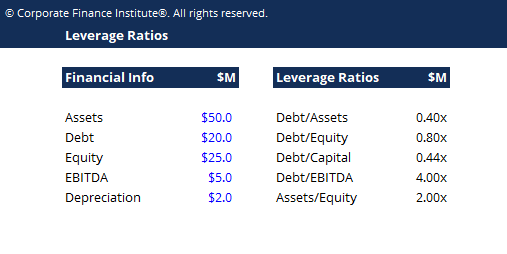# Leverage Ratios Template

## Leverage Ratios Template

This leverage ratios template helps you calculate five commonly used leverage ratios.

Below is a preview of the leverage ratios template:### Leverage Ratios Template

A leverage ratio is any kind of financial ratio that indicates the level of debt incurred by a business entity against several other accounts in its balance sheetincome statement, or cash flow statement.  These ratios provide an indication of how the company’s assets and business operations are financed (using debt or equity).

Here are five of the most commonly used leverage ratios:

1. Debt-to-Assets Ratio = Total Debt / Total Assets

2. Debt-to-Equity Ratio = Total Debt / Total Equity

3. Debt-to-Capital Ratio = Today Debt / (Total Debt + Total Equity)

4. Debt-to-EBITDA Ratio = Total Debt / Earnings Before Interest Taxes Depreciation & Amortization (EBITDA)

5. Asset-to-Equity Ratio = Total Assets / Total Equity

### More Free Templates

For more resources, check out our business templates library to download numerous free Excel modeling, PowerPoint presentation and Word document templates.

• Excel Modeling Templates
• PowerPoint Presentation Templates
• Transaction Document Templates

### Financial Analyst Certification

Become a certified Financial Modeling and Valuation Analyst (FMVA)® by completing CFI’s online financial modeling classes and training program!